# How to Average Absolute Values in Excel

We can use AVERAGE function to calculate average of certain values. We can use ABS function to get absolute values for both positive number and negative number. If we want to get the average absolute values, we need to combine both above two functions in the formula. In this free tutorial, we will provide two formulas for you to average absolute values.

Precondition:

Prepare table below. There are some negative numbers.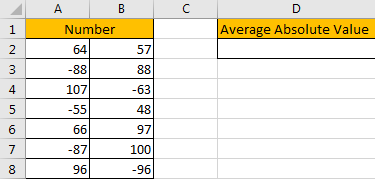## Method: Average Absolute Values by Formula

Step 1: In D2 enter the formula =AVERAGE(ABS(A2:B8)).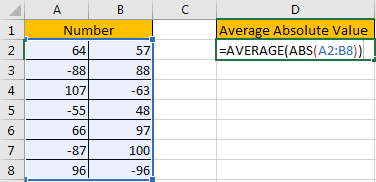Step 2: As it is an array formula, so press Ctrl+Shift+Enter to get value.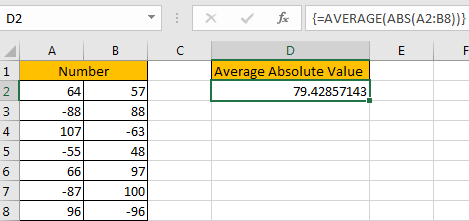Step 3: If you don’t want to enter an array formula and you like apply a regular formula instead, you can enter this formula =AVERAGE(INDEX(ABS(A2:B8),0,0)).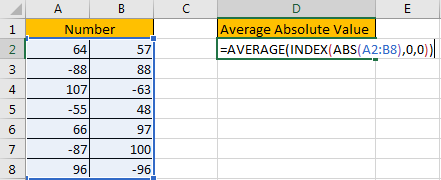Step 4: Just press Enter as usual.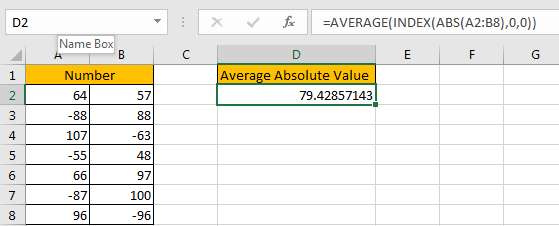We get the same result.

### Related Functions

• Excel AVERAGE function
The Excel AVERAGE function returns the average of the numbers that you provided.The syntax of the AVERAGE function is as below:=AVERAGE (number1,[number2],…)….
• Excel ABS Function
The Excel ABS function returns the absolute value of a number.The ABS function is a build-in function in Microsoft Excel and it is categorized as a Math and Trigonometry Function.The syntax of the ABS function is as below:=ABS (number)…
• Excel INDEX function
The Excel INDEX function returns a value from a table based on the index (row number and column number)The INDEX function is a build-in function in Microsoft Excel and it is categorized as a Lookup and Reference Function.The syntax of the INDEX function is as below:= INDEX (array, row_num,[column_num])…
Related Posts

Break ties with helper COUNTIF and column

Suppose you got a task to adjust the values that contain the ties; what would be your first attempt to break the ties of the given value? If you are wondering about doing this task manually, let me add that ...

Find the Closest Data to the Data Provided in Excel

In our daily work, we may encounter such an issue that to find the closest value to a certain value. In fact, Excel internal functions can help us solve this problem. In today’s article, we will show you how to ...

Creating a Table with Automatic Row Numbering

When creating an Excel Table, you may use a calculation relying on the ROW function to insert row numbers automatically. The formula in A2, which has been copied down, is as follows: =ROW()-ROW(Table1[#Headers]) Note: the default table name is Table1. ...

Get Address of First Cell in Range

We will learn how to get address of first cell in range in this post. At Instance, we must determine the address of first cell in range to use as the data table's value. For the DATA table, we have ...

Convert State Names To Abbreviations

Assume that you got a task to convert the full state's name into the abbreviations in MS Excel, and for doing this task, you might choose to do it manually, which is an acceptable way only if you don't have ...

Filter or Extract with a Partial Match

Assume you have a table consisting of a few cells having few values, and you want to filter out the set of records with the partial match. You might take it easy and would prefer to manually filter out the ...

Find and Replace Multiple Values

This post will guide you how to find and replace multiple values at once with VBA macro or using formula  in Excel. How do I make multiple find and replace in Excel. Suppose that you have a few cells containing ...

Excel XLOOKUP Function

Excel XLOOKUP Function was added into Excel as a beta feature in August 2019 and is now accessible exclusively in Microsoft 365. (as of July 2021). However, if you fall into this category and often deal with big sets of ...

Extract or Filter Top n values

You might have been through this kind of situation where you need to filter out the top n values from the list having few values, and I am also pretty sure about it that you might have chosen to do ...

Extract matching values From Two Lists

Suppose that you are working with two lists containing few values, and you want to extract the matching values from those two lists into another separate list. You might prefer to manually extract the matching values from the two lists, ...

Sidebar